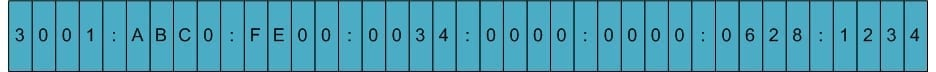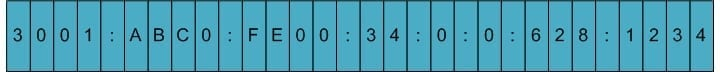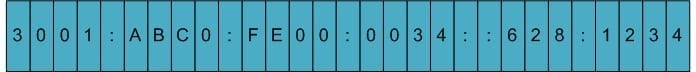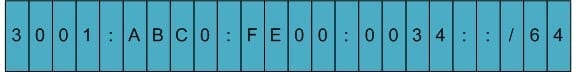## Overview

When learning IPv6 addressing, one of the first things that people notice is that it is much more complex to look at than the previous IPv4 addresses; this is compounded by the fact that a single address can be notated in a number of different ways and still refers to the same device. This article takes a look at IPv6 notation, how a single IPv6 address can be notated in a number of different ways, and how to expand and compress them correctly.

An IPv6 address has a total of 128 bits that are represented in hexadecimal form, using 8 – 4 hex character groupings.  Figure 1 below shows a fully expanded IPv6 address:Figure 1 – Expanded IPv6 Address

There are two ways that an IPv6 address can be additionally compressed: compressing leading zeros and substituting a group of consecutive zeros with a single double colon (::). Both of these can be used in any number of combinations to notate the same address. It is important to note that the double colon (::) can only be used once within a single IPv6 address notation. Figure 2 below shows what the same address shown in Figure 1 would look like if the leading zeros were removed.Figure 2 – Compressing Leading Zeros
Another method is to use the double colon (::).  Figure 3 shows how the use of a double colon (::) could be used to compress the IPv6 address:Figure 3 – Compressing using Double Colon (::)
The final compression method removes the leading zeros and uses the double colon (::) to provide the shortest IPv6 notation. Figure 4 shows what this completely compressed IPv6 address would look like:Figure 4 – Compressing Leading Zeros and using Double Colon (::)